### NCERT Solutions For Class 9 Math Chapter – 13 Exercise – 13.2

NCERT Solutions For Class 9 Math Chapter – 13 Exercise – 13.2

Q1.  The curved surface area of a right circular cylinder of height 14 cm is 88 cm2. Find the diameter of the base of the cylinder.

Solution:-

Curved surface area of cylinder = 2πrh

= 2πrh = 88

= 2 x 22/7 x r x 14 = 88

R = 88 x 7/2 x 22 x 14

R = 1 cm

Diameter = 2 x r

= (2 x 1 ) cm

= 2 cm

Q2. It is required to make a closed cylindrical tank of height 1 m and base diameter 140 cm from a meta sheet How man square meters of the sheet are required for the same?

Solution:-

Total

surface area of cylinder = 2πr(h+r)

= 2 x 22/7 x 0.70 (1+0.70) m2

= 2 x 22 x 0.10 x 1.70 m2

= 7.48 m2.

Hence the required sheet = 7.48 m2.

Q3. A metal pipe is 77 cm long The inner ft diameter of a cross section is 4 cm, the

outer diameter being 4.4cm. (see fig. 13.11). Find its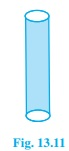(i) inner curved surface area,

(ii) outer curved surface area

(iii) total surface area

(Assume π=22/7)

Solution:

Let r1 and r2 Inner and outer radii of cylindrical pipe

r= 4/2 cm = 2 cm

r= 4.4/2 cm = 2.2 cm

Height of cylindrical pipe, h = length of cylindrical pipe = 77 cm

(i) curved surface area of outer surface of pipe = 2πr1h

= 2×(22/7)×2×77 cm2

= 968 cm2

(ii) curved surface area of outer surface of pipe = 2πr2h

= 2×(22/7)×2.2×77 cm2

= (22×22×2.2) cm2

= 1064.8 cm2

(iii) Total surface area of pipe = inner curved surface area+ outer curved surface area+ Area of both circular ends of pipe.

= 2r1h+2r2h+2π(r12-r22)

= 9668+1064.8+2×(22/7)×(2.22-22)

= 2031.8+5.28

= 2038.08 cm2

Therefore, the total surface area of the cylindrical pipe is 2038.08 cm2.

4. The diameter of a roller is 84 cm and its length is 120 cm. It takes 500 complete revolutions to

move once over to level a playground. Find the area of the playground in m2? (Assume π = 22/7)

Solution:

A roller is shaped like a cylinder.

Let h be the height of the roller and r be the radius.

h = Length of roller = 120 cm

Radius of the circular end of roller = r = (84/2) cm = 42 cm

Now, CSA of roller = 2πrh

= 2×(22/7)×42×120

= 31680 cm2

Area of field = 500×CSA of roller

= (500×31680) cm2

= 15840000 cm2

= 1584 m2.

Therefore, area of playground is 1584 m2. Answer!

5. A cylindrical pillar is 50 cm in diameter and 3.5 m in height. Find the cost of painting the curved surface of the pillar at the rate of Rs. 12.50 per m2.

(Assume π = 22/7)

Solution:

Let h be the height of a cylindrical pillar and r be the radius.

Given:

Height cylindrical pillar = h = 3.5 m

Radius of the circular end of pillar = r = diameter/2 = 50/2 = 25cm = 0.25m

CSA of pillar = 2πrh

= 2×(22/7)×0.25×3.5

= 5.5 m2

Cost of painting 1 m2 area = Rs. 12.50

Cost of painting 5.5 m2 area = Rs (5.5×12.50)

= Rs.68.75

Therefore, the cost of painting the curved surface of the pillar at the rate of Rs. 12.50 per m2 is Rs 68.75.

6. Curved surface area of a right circular cylinder is 4.4 m2. If the radius of the base of the base of the cylinder is 0.7 m, find its height. (Assume π = 22/7)

Solution:

Let h be the height of the circular cylinder and r be the radius.

Radius of the base of cylinder, r = 0.7m

CSA of cylinder = 2πrh

CSA of cylinder = 4.4m2

Equating both the equations, we have

2×(22/7)×0.7×h = 4.4

Or h = 1

Therefore, the height of the cylinder is 1 m.

7. The inner diameter of a circular well is 3.5m. It is 10m deep. Find

(i) its inner curved surface area,

(ii) the cost of plastering this curved surface at the rate of Rs. 40 per m2.

(Assume π = 22/7)

Solution:

Inner radius of circular well, r = 3.5/2m = 1.75m

Depth of circular well, say h = 10m

(i) Inner curved surface area = 2πrh

= (2×(22/7 )×1.75×10)

= 110

Therefore, the inner curved surface area of the circular well is 110 m2.

(ii)Cost of plastering 1 m2 area = Rs.40

Cost of plastering 110 m2 area = Rs (110×40)

= Rs.4400

Therefore, the cost of plastering the curved surface of the well is Rs. 4400.

8. In a hot water heating system, there is cylindrical pipe of length 28 m and diameter 5 cm. Find

the total radiating surface in the system. (Assume π = 22/7)

Solution:

Height of cylindrical pipe = Length of cylindrical pipe = 28m

Radius of circular end of pipe = diameter/ 2 = 5/2 cm = 2.5cm = 0.025m

Now, CSA of cylindrical pipe = 2πrh, where r = radius and h = height of the cylinder

= 2×(22/7)×0.025×28 m2

= 4.4m2

The area of the radiating surface of the system is 4.4m2.

9. Find

(i) the lateral or curved surface area of a closed cylindrical petrol storage tank that is 4.2 m in

diameter and 4.5m high.

(ii) How much steel was actually used, if 1/12 of the steel actually used was wasted in making the tank. (Assume π = 22/7)

Solution:

Height of cylindrical tank, h = 4.5m

Radius of the circular end , r = (4.2/2)m = 2.1m

(i) the lateral or curved surface area of cylindrical tank is 2πrh

= 2×(22/7)×2.1×4.5 m2

= (44×0.3×4.5) m2

= 59.4 m2

Therefore, CSA of tank is 59.4 m2.

(ii) Total surface area of tank = 2πr(r+h)

= 2×(22/7)×2.1×(2.1+4.5)

= 44×0.3×6.6

= 87.12 m2

Now, Let S m2 steel sheet be actually used in making the tank.

S(1 -1/12) = 87.12 m2

This implies, S = 95.04 m2

Therefore, 95.04msteel was used in actual while making such a tank.

10. In fig. 13.12, you see the frame of a lampshade. It is to be covered with a decorative cloth.

The frame has a base diameter of 20 cm and height of 30 cm. A margin of 2.5 cm is to be given for folding it over the top and bottom of the frame. Find how much cloth is required for covering the lampshade. (Assume π = 22/7)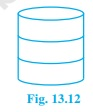Solution:

Say h = height of the frame of lampshade, looks like cylindrical shape

Total height is h = (2.5+30+2.5) cm = 35cm and

r = (20/2) cm = 10cm

Use curved surface area formula to find the cloth required for covering the lampshade which is 2πrh

= (2×(22/7)×10×35) cm2

= 2200 cm2

Hence, 2200 cm2 cloth is required for covering the lampshade.

11. The students of Vidyalaya were asked to participate in a competition for making and decorating penholders in the shape of a cylinder with a base, using cardboard. Each penholder was to be of radius 3 cm and height 10.5 cm. The Vidyalaya was to supply the competitors with cardboard. If there were 35 competitors, how much cardboard was required to be bought for the competition? (Assume π =22/7)

Solution:

Radius of the circular end of cylindrical penholder, r = 3cm

Height of penholder, h = 10.5cm

Surface area of a penholder = CSA of pen holder + Area of base of penholder

= 2πrh+πr2

= 2×(22/7)×3×10.5+(22/7)×32= 1584/7

Therefore, Area of cardboard sheet used by one competitor is 1584/7 cm2

So, Area of cardboard sheet used by 35 competitors = 35×1584/7 = 7920 cm2

Therefore, 7920 cm2 cardboard sheet will be needed for the competition.

## Exercise 13.3 Page No: 221

1. Diameter of the base of a cone is 10.5 cm and its slant height is 10 cm. Find its curved surface area (Assume π=22/7)

Solution:

Radius of the base of cone = diameter/ 2 = (10.5/2)cm = 5.25cm

Slant height of cone, say l = 10 cm

CSA of cone is = πrl

= (22/7)×5.25×10 = 165

Therefore, the curved surface area of the cone is 165 cm2.

2. Find the total surface area of a cone, if its slant height is 21 m and diameter of its base is 24 m. (Assume π = 22/7)

Solution:

Radius of cone, r = 24/2 m = 12m

Slant height, l = 21 m

Formula: Total Surface area of the cone = πr(l+r)

Total Surface area of the cone = (22/7)×12×(21+12) m2

= 1244.57m2

3. Curved surface area of a cone is 308 cm2 and its slant height is 14 cm. Find

(i) radius of the base and (ii) total surface area of the cone.

(Assume π = 22/7)

Solution:

Slant height of cone, l = 14 cm

Let the radius of the cone be r.

(i) We know, CSA of cone = πrl

Given: Curved surface area of a cone is 308 cm2

(308 ) = (22/7)×r×14

308 = 44 r

r = 308/44 = 7

Radius of a cone base is 7 cm.

(ii) Total surface area of cone = CSA of cone + Area of base (πr2)

Total surface area of cone = 308+(22/7)×72 = 308+154

Therefore, the total surface area of the cone is 462 cm2.

4. A conical tent is 10 m high and the radius of its base is 24 m. Find

(i) slant height of the tent.

(ii) cost of the canvas required to make the tent, if the cost of 1 m2 canvas is Rs 70.

(Assume π=22/7)

Solution: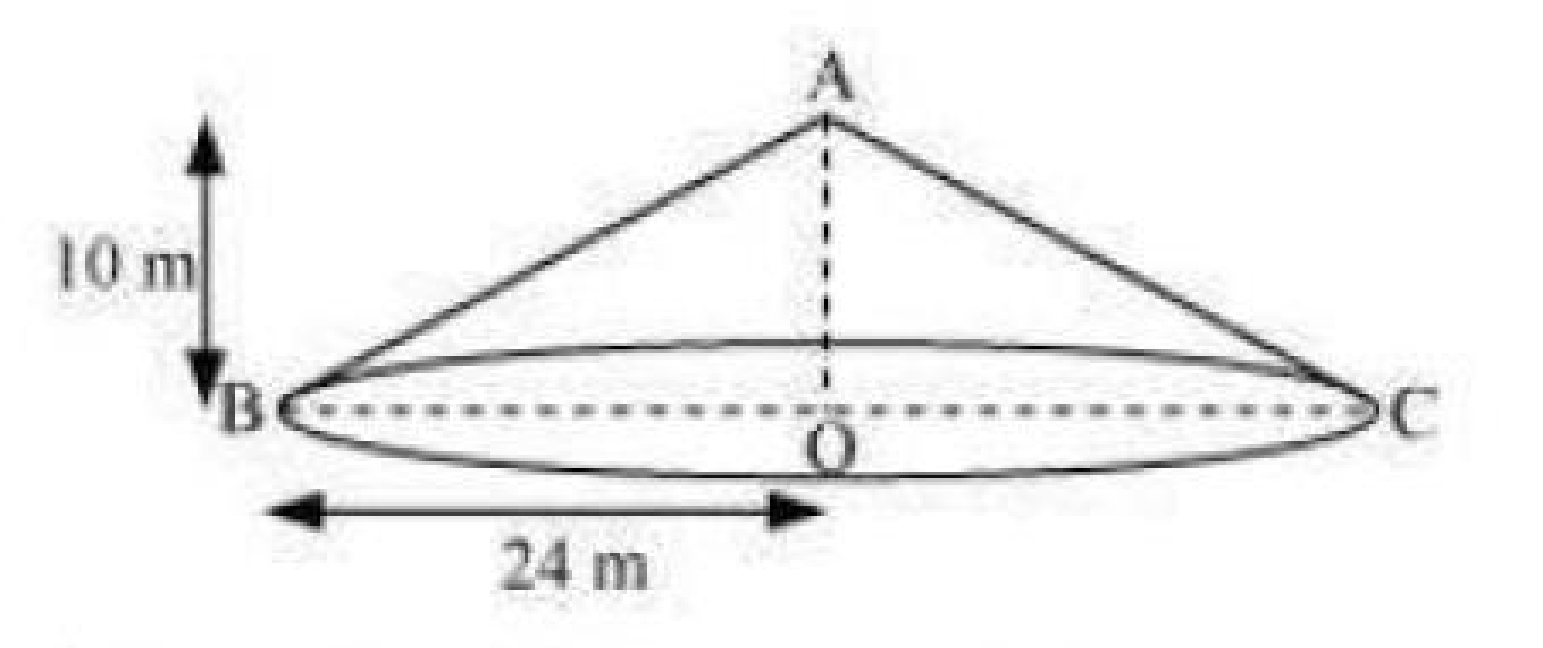Let ABC be a conical tent

Height of conical tent, h = 10 m

Radius of conical tent, r = 24m

Let the slant height of the tent be l.

(i) In right triangle ABO, we have

AB= AO2+BO2(using Pythagoras theorem)

l2 = h2+r2

= (10)2+(24)2

= 676

l = 26

Therefore, the slant height of the tent is 26 m.

(ii) CSA of tent = πrl

= (22/7)×24×26 m2

Cost of 1 m2 canvas = Rs 70

Cost of (13728/7)m2 canvas is equal to Rs (13728/7)×70 = Rs 137280

Therefore, the cost of the canvas required to make such a tent is Rs 137280.

5. What length of tarpaulin 3 m wide will be required to make conical tent of height 8 m and base radius 6m? Assume that the extra length of material that will be required for stitching margins and wastage in cutting is approximately 20 cm. [Use π=3.14]

Solution:

Height of conical tent, h = 8m

Radius of base of tent, r = 6m

Slant height of tent, l2 = (r2+h2)

l= (62+82) = (36+64) = (100)

or l = 10

Again, CSA of conical tent = πrl

= (3.14×6×10) m2

= 188.4m2

Let the length of tarpaulin sheet required be L

As 20 cm will be wasted, therefore,

Effective length will be (L-0.2m).

Breadth of tarpaulin = 3m (given)

Area of sheet = CSA of tent

[(L–0.2)×3] = 188.4

L-0.2 = 62.8

L = 63

Therefore, the length of the required tarpaulin sheet will be 63 m.

6. The slant height and base diameter of conical tomb are 25m and 14 m respectively. Find the cost of white-washing its curved surface at the rate of Rs. 210 per 100 m2. (Assume π = 22/7)

Solution:

Slant height of conical tomb, l = 25m

Base radius, r = diameter/2 = 14/2 m = 7m

CSA of conical tomb = πrl

= (22/7)×7×25 = 550

CSA of conical tomb= 550m2

Cost of white-washing 550 m2 area, which is Rs (210×550)/100

= Rs. 1155

Therefore, cost will be Rs. 1155 while white-washing tomb.

7. A joker’s cap is in the form of right circular cone of base radius 7 cm and height 24cm. Find the area of the sheet required to make 10 such caps. (Assume π =22/7)

Solution:

Radius of conical cap, r = 7 cm

Height of conical cap, h = 24cm

Slant height, l2 = (r2+h2)

= (72+242)

= (49+576)

= (625)

Or l = 25 cm

CSA of 1 conical cap = πrl

= (22/7)×7×25

= 550

CSA of 10 caps = (10×550) cm2 = 5500 cm2

Therefore, the area of the sheet required to make 10 such caps is 5500 cm2.

8. A bus stop is barricaded from the remaining part of the road, by using 50 hollow cones made of recycled cardboard. Each cone has a base diameter of 40 cm and height 1 m. If the outer side of each of the cones is to be painted and the cost of painting is Rs. 12 per m2, what will be the cost of painting all these cones? (Use π = 3.14 and take √(1.04) =1.02)

Solution:

Given:

Radius of cone, r = diameter/2 = 40/2 cm = 20cm = 0.2 m

Height of cone, h = 1m

Slant height of cone is l, and l= (r2+h2)

Using given values, l2 = (0.22+12)

= (1.04)

Or l = 1.02

Slant height of the cone is 1.02 m

Now,

CSA of each cone = πrl

= (3.14×0.2×1.02)

= 0.64056

CSA of 50 such cones = (50×0.64056) = 32.028

CSA of 50 such cones = 32.028 m2

Again,

Cost of painting 1 m2 area = Rs 12 (given)

Cost of painting 32.028 m2 area = Rs (32.028×12)

= Rs.384.336

= Rs.384.34 (approximately)

Therefore, the cost of painting all these cones is Rs. 384.34.

## Exercise 13.4 Page No: 225

1. Find the surface area of a sphere of radius:

(i) 10.5cm (ii) 5.6cm (iii) 14cm

(Assume π=22/7)

Solution:

Formula: Surface area of sphere (SA) = 4πr2

(i) Radius of sphere, r = 10.5 cm

SA = 4×(22/7)×10.5= 1386

Surface area of sphere is 1386 cm2

(ii) Radius of sphere, r = 5.6cm

Using formula, SA = 4×(22/ 7)×5.6= 394.24

Surface area of sphere is 394.24 cm2

(iii) Radius of sphere, r = 14cm

SA = 4πr2

= 4×(22/7)×(14)2

= 2464

Surface area of sphere is 2464 cm2

2. Find the surface area of a sphere of diameter:

(i) 14cm (ii) 21cm (iii) 3.5cm

(Assume π = 22/7)

Solution:

(i) Radius of sphere, r = diameter/2 = 14/2 cm = 7 cm

Formula for Surface area of sphere = 4πr2

= 4×(22/7)×72 = 616

Surface area of a sphere is 616 cm2

(ii) Radius (r) of sphere = 21/2 = 10.5 cm

Surface area of sphere = 4πr2

= 4×(22/7)×10.5= 1386

Surface area of a sphere is 1386 cm2

Therefore, the surface area of a sphere having diameter 21cm is 1386 cm2

(iii) Radius(r) of sphere = 3.5/2 = 1.75 cm

Surface area of sphere = 4πr2

= 4×(22/7)×1.752 = 38.5

Surface area of a sphere is 38.5 cm2

3. Find the total surface area of a hemisphere of radius 10 cm. [Use π=3.14]

Solution:

Radius of hemisphere, r = 10cm

Formula: Total surface area of hemisphere = 3πr2

= 3×3.14×102 = 942

The total surface area of given hemisphere is 942 cm2.

4. The radius of a spherical balloon increases from 7cm to 14cm as air is being pumped into it. Find the ratio of surface areas of the balloon in the two cases.

Solution:

Let rand r2 be the radii of spherical balloon and spherical balloon when air is pumped into it respectively. So

r= 7cm

r= 14 cm

Now, Required ratio = (initial surface area)/(Surface area after pumping air into balloon)

= 4r12/4r22

= (r1/r2)2

= (7/14)= (1/2)2 = ¼

Therefore, the ratio between the surface areas is 1:4.

5. A hemispherical bowl made of brass has inner diameter 10.5cm. Find the cost of tin-plating it on the inside at the rate of Rs 16 per 100 cm2. (Assume π = 22/7)

Solution:

Inner radius of hemispherical bowl, say r = diameter/2 = (10.5)/2 cm = 5.25 cm

Formula for Surface area of hemispherical bowl = 2πr2

= 2×(22/7)×(5.25)2 = 173.25

Surface area of hemispherical bowl is 173.25 cm2

Cost of tin-plating 100 cm2 area = Rs 16

Cost of tin-plating 1 cm2 area = Rs 16 /100

Cost of tin-plating 173.25 cmarea = Rs. (16×173.25)/100 = Rs 27.72

Therefore, the cost of tin-plating the inner side of the hemispherical bowl at the rate of Rs 16 per 100 cm2 is Rs 27.72.

6. Find the radius of a sphere whose surface area is 154 cm2. (Assume π = 22/7)

Solution:

Let the radius of the sphere be r.

Surface area of sphere = 154 (given)

Now,

4πr= 154

r= (154×7)/(4×22) = (49/4)

r = (7/2) = 3.5

The radius of the sphere is 3.5 cm.

7. The diameter of the moon is approximately one fourth of the diameter of the earth.

Find the ratio of their surface areas.

Solution:

If diameter of earth is said d, then the diameter of moon will be d/4 (as per given statement)

Radius of moon = ½×d/4 = d/8

Surface area of moon = 4π(d/8)2

Surface area of earth = 4π(d/2)2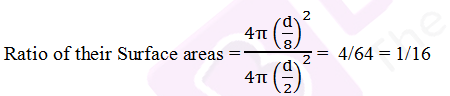The ratio between their surface areas is 1:16.

8. A hemispherical bowl is made of steel, 0.25 cm thick. The inner radius of the bowl is 5cm. Find the outer curved surface of the bowl. (Assume π =22/7)

Solution:

Given:

Inner radius of hemispherical bowl = 5cm

Thickness of the bowl = 0.25 cm

Outer radius of hemispherical bowl = (5+0.25) cm = 5.25 cm

Formula for outer CSA of hemispherical bowl = 2πr2, where r is radius of hemisphere

= 2×(22/7)×(5.25)2 = 173.25

Therefore, the outer curved surface area of the bowl is 173.25 cm2.

9. A right circular cylinder just encloses a sphere of radius r (see fig. 13.22). Find

(i) surface area of the sphere,

(ii) curved surface area of the cylinder,

(iii) ratio of the areas obtained in(i) and (ii).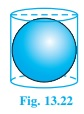Solution:

(i) Surface area of sphere = 4πr2, where r is the radius of sphere

(ii) Height of cylinder, h = r+r =2r